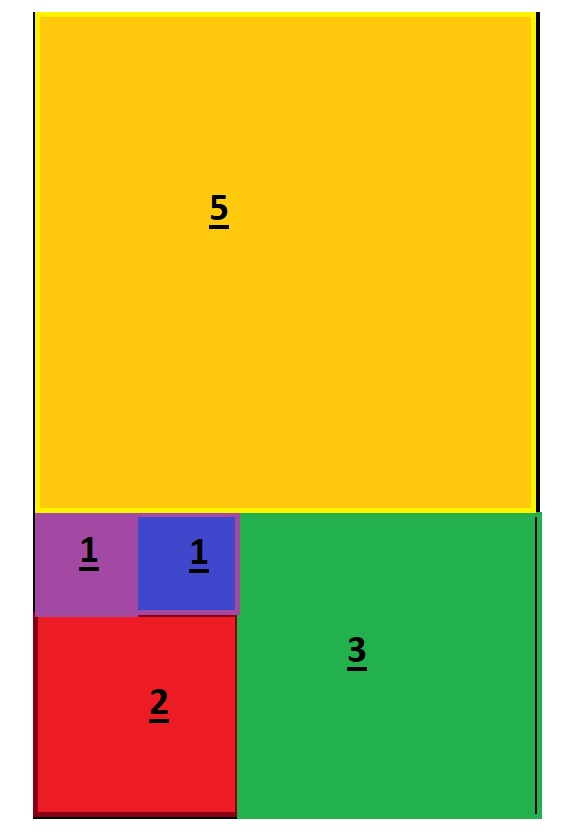# Sum of perimeter of all the squares in a rectangle using JavaScript

JavascriptWeb DevelopmentFront End Technology

Problem Suppose there are 5 squares embedded inside a rectangle like this −Their perimeter will be −

4 + 4 + 8 + 12 + 20 = 48 units

We are required to write a JavaScript function that takes in a number n and return the sum of the perimeter if there are n squares embedded.

## Example

Following is the code −

Live Demo

const num = 6;
const findPerimeter = (num = 1) => {
const arr = [1,1];
let n = 0;
let sum = 2;
for(let i = 0 ; i < num-1 ; i++){
n = arr[i] + arr[i+1];
arr.push(n);
sum += n;
};
return sum * 4;
};
console.log(findPerimeter(num - 1));

## Output

80